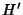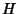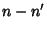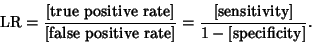## Likelihood Ratio

A quantity used to test Nested Hypotheses. Letbe a Nested Hypothesis withDegrees of Freedom within(which hasDegrees of Freedom), then calculate the Maximum Likelihood of a given outcome, first given, then given. ThenComparison of this ratio to the critical value of the Chi-Squared Distribution withDegrees of Freedom then gives the Significance of the increase in Likelihood.

The term likelihood ratio is also used (especially in medicine) to test nonnested complementary hypotheses as follows,